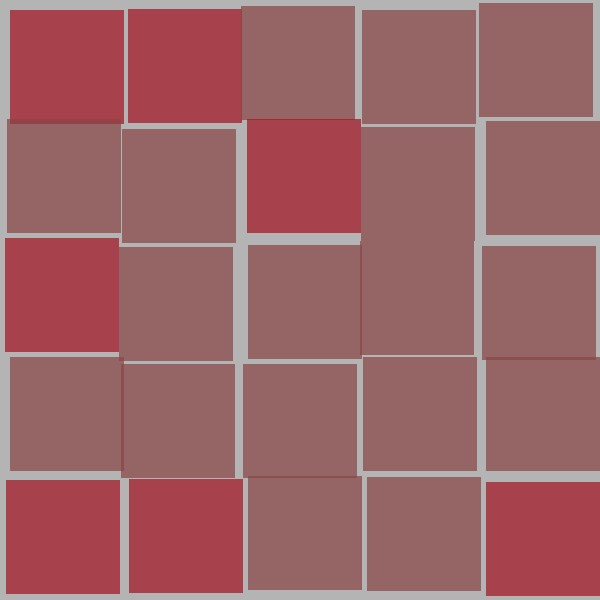JRubyArt and propane both have this convenience method `grid` that seldom gets used

``````# A nice method to run a given block for a grid.
# Lifted from action_coding/Nodebox.
def grid(cols, rows, col_size = 1, row_size = 1)
(0...cols * rows).map do |i|
x = col_size * (i % cols)
y = row_size * i.div(cols)
yield x, y
end
end

``````

But we definitely should use it to simplify the coding of grids as below

``````# Creative Coding
# # # # #
# Vera Molnar – 25 Squares
# # # # #
# Interpretation by Martin Vögeli
# Converted to propane Martin Prout
# # # # #
# Based on code by Indae Hwang and Jon McCormack
def settings
size(600, 600)
end

def setup
sketch_title '25 Squares'
rect_mode(CORNER)
no_stroke
frame_rate(1) # set the frame rate to 1 draw call per second
end

def draw
background(180) # clear the screen to grey
grid_size = 5 # rand(3..12)   # select a rand number of squares each frame
gap = 5 # rand(5..50) # select a rand gap between each square
# calculate the size of each square for the given number of squares and gap between them
cellsize = (width - (grid_size + 1) * gap) / grid_size
position = -> (count) { gap * (count + 1) + cellsize * count + rand(-5..5) }
grid(grid_size, grid_size) do |x, y|
rand(0..5) > 4 ? fill(color('#a11220'), 180.0) : fill(color('#884444'), 180.0)
rect(position.call(x), position.call(y), cellsize, cellsize)
end
# save your drawings when you press keyboard 's' continually
save_frame('######.jpg') if key_pressed? && key == 's'
end # end of draw
``````